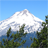# Floating split

225
3
09-19-2017 05:28 AMNew Contributor III

Hello

I'm trying to split a surface into as many parts as possible without any leftover space, so I figured that the floating split would be perfect. Unfortunately, I encounter a problem where the split distance is shorter than the floating number, is there a way to make the floating number the minimum distance?

An excample could be that you have an 11 meters long surface you need to split into smaller surfaces with the floating distance of 2. I would then write:

Surface -->

split(x) {~2 : Surface01 }*

in this case, the split can produce either 5 splits that each is 2.2 meters or 6 splits that are 1.83 meters, I guess that CE would create 6 splits as that creates the width that is closest to 2, but what would if I wanted it to do five splits?. Is there a way to ensure that 2 is a minimum distance?

Kind regards

Kristian

Tags (1)
3 Repliesby
Regular Contributor

Repeat splits fix the leftover automatically. Just do hard 2m splits, that way you'll end up with 5x2m and and a 1m leftover. You can also nest repeat splits, like so: split(x){~1: X. | {2: X.}* | ~1: X.}.New Contributor III

Thanks for the answer and your solutions would work in the specific case, but it is not directly what I'm looking for, the problem is I don't want to have any "leftover" space at the edges but have it divided into equal sizes with a minimum distance of 2, everything above 2 is fine.by
Regular Contributor

Ah. Then all you need is a little math.

``split(x){(minWidth+((minWidth*((scope.sx/minWidth)-(floor(scope.sx/minWidth))))/(floor(scope.sx/minWidth)))): X}*‍``